## Analogy

#### Analogy

Direction: In each of the following questions select the related figure from the given alternatives.

1. Find the question mark ? figure from answer figure.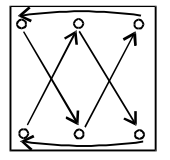1. From first figure to second figure following changes occur: Similar changes would occur in the second pair of figures.

##### Correct Option: B

From first figure to second figure following changes occur: Similar changes would occur in the second pair of figures.

1. Find the question mark ? figure from answer figure.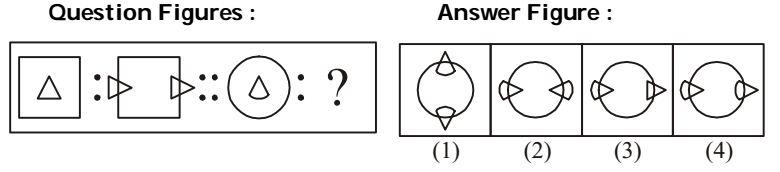1. From first unit to second unit the triangle rotates through 90° clockwise and moves to the side of square and a similar triangle appears on the opposite side.

##### Correct Option: D

From first unit to second unit the triangle rotates through 90° clockwise and moves to the side of square and a similar triangle appears on the opposite side.

1. Find the question mark ? figure from answer figure.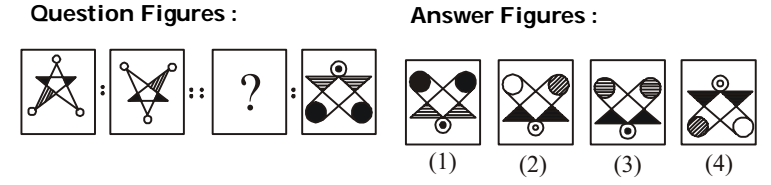1. The second figure in the first unit of Problem Figure can be obtained by rotating the first figure by 180°.

##### Correct Option: A

The second figure in the first unit of Problem Figure can be obtained by rotating the first figure by 180°.

1. Find the question mark ? figure from answer figure.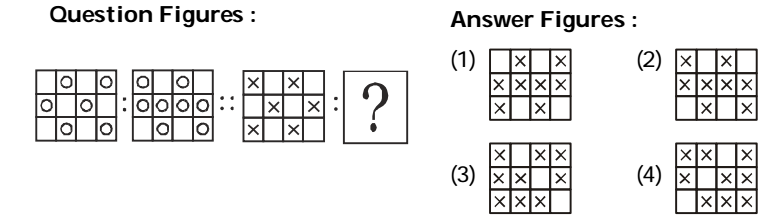1. From first figure to second figure the circles of upper row move one block in anticlockwise direction and two circles are added in the middle row. In similar way, the two crosses of lower row move one block in anticlockwise direction and two crosses are added in the middle row.

##### Correct Option: B

From first figure to second figure the circles of upper row move one block in anticlockwise direction and two circles are added in the middle row. In similar way, the two crosses of lower row move one block in anticlockwise direction and two crosses are added in the middle row.

1. Find the question mark ? figure from answer figure.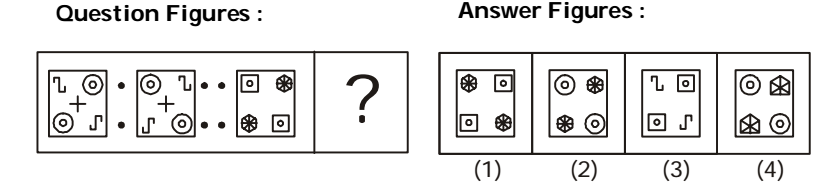1. From first figure to second figure, four designs move one step in clockwise direction.

##### Correct Option: A

From first figure to second figure, four designs move one step in clockwise direction.